Courses

# Boundary Value Problems(Part- 2) - Electrostatics, Electromagnetic Theory, CSIR-NET Physical Science Physics Notes | EduRev

## Physics for IIT JAM, UGC - NET, CSIR NET

Created by: Akhilesh Thakur

## Physics : Boundary Value Problems(Part- 2) - Electrostatics, Electromagnetic Theory, CSIR-NET Physical Science Physics Notes | EduRev

The document Boundary Value Problems(Part- 2) - Electrostatics, Electromagnetic Theory, CSIR-NET Physical Science Physics Notes | EduRev is a part of the Physics Course Physics for IIT JAM, UGC - NET, CSIR NET.
All you need of Physics at this link: Physics

Example: The Electrostatic Fields of a Coaxial Line

A common form of a transmission line is the coaxial cable.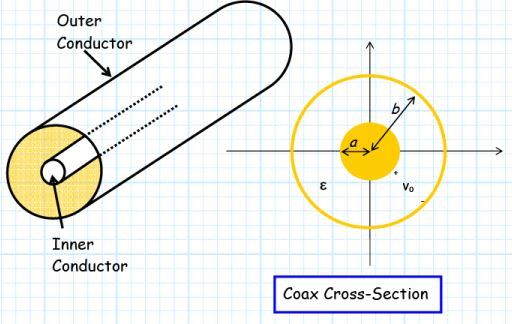The coax has an outer diameter b, and an inner diameter a.
The space between the conductors is filled with dielectric material of permittivity ε .

Say a voltage V0 is placed across the conductors, such that the electric potential of the outer conductor is zero, and the electric potential of the inner conductor is V0.

The potential difference between the inner and outer conductor is therefore V0 – 0 = Vvolts.

Q: What electric potential field V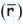, electric field Eand charge density ρsis produced by this situation?

A: We must solve a boundary-value problem!  We must find solutions that:

a) Satisfy the differential equations of electrostatics (e.g., Poisson’s, Gauss’s).

b)  Satisfy the electrostatic boundary conditions.

Yikes! Where do we start ?

We might start with the electric potential field V, since it is a scalar field.

a)  The electric potential function must satisfy Poisson’s equation: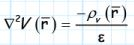b)  It must also satisfy the boundary conditions:

V (ρ = a) =V            V (ρ = b) = 0

Consider first the dielectric region (a < ρ < b ).  Since the region is a dielectric, there is no free charge, and:

ρv=0

Therefore, Poisson’s equation reduces to Laplace’s equation: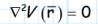This particular problem (i.e., coaxial line) is directly solvable because the structure is cylindrically symmetric.  Rotating the coax around the z-axis (i.e., in the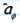direction) does not change the geometry at all. As a result, we know that the electric potential field is a function of ρ only ! I.E.,:

V= V ( ρ )
This make the problem much easier.  Laplace’s equation becomes: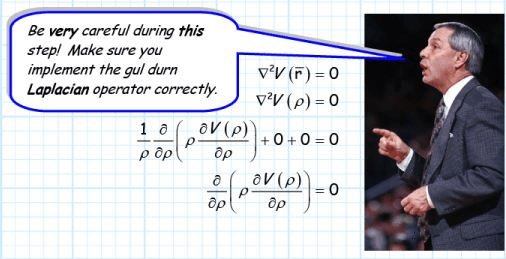Integrating both sides of the resulting equation, we find: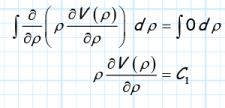where C1 is some constant.

Rearranging the above equation, we find: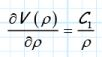Integrating both sides again, we get: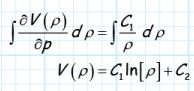We find that this final equation (V ( ρ ) = C 1 ln [ρ ] +C) will satisfy Laplace’s equation  (try it!).

We must now apply the boundary conditions to determine the value of constants C1 and C2.

• We know that on the outer surface of the inner conductor (i.e., ρ = a ), the electric potential is equal to V0 (i.e., V ( ρ = a)=V 0 ).
•  And, we know that on the inner surface of the outer conductor (i.e., ρ = b ) the electric potential is equal to zero (i.e., V ( ρ = b)= 0 ).

Therefore, we can write: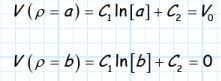Two equations and two unknowns (Cand C2)!

Solving for C1 and C2 we get: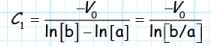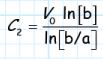and therefore, the electric potential field within the dielectric is found to be: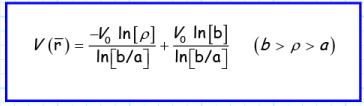Before we move on, we should do a sanity check to make sure we have done everything correctly.  Evaluating our result at ρ = a , we get: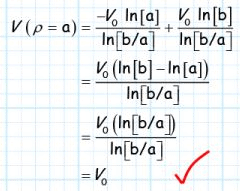Likewise, we evaluate our result at ρ = b :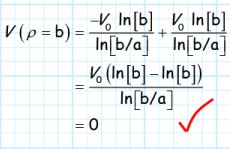Our result is correct!

Now, we can determine the electric field within the dielectric by taking the gradient of the electric potential field: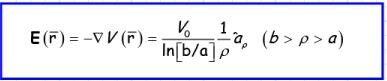Note that electric flux density is therefore: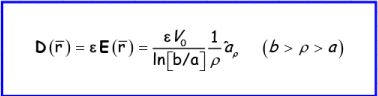Finally, we need to determine the charge density that actually created these fields!

Q1: Just where is this charge? After all, the dielectric (if it is perfect) will contain no free charge.

A1:  The free charge, as we might expect, is in the conductors.  Specifically, the charge is located at the surface of the conductor.

Q2: Just how do we determine this surface charge ρs?

A2: Apply the boundary conditions!

Recall that we found that at a conductor/dielectric interface, the surface charge density on the conductor is related to the electric flux density in the dielectric as: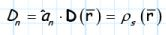First, we find that the electric flux density on the surface of the inner conductor (i.e., at ρ = a ) is: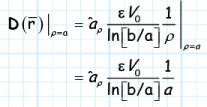For every point on outer surface of the inner conductor, we find that the unit vector normal to the conductor is: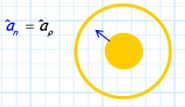Therefore, we find that the surface charge density on the outer surface of the inner conductor is: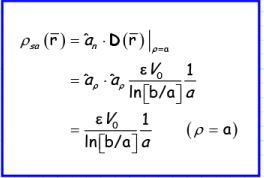Likewise, we find the unit vector normal to the inner surface of the outer conductor is (do you see why?):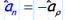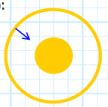Therefore, evaluating the electric flux density on the inner surface of the outer conductor (i.e., ρ = b ), we find: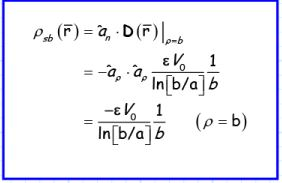Note the charge on the outer conductor is negative, while that of the inner conductor is positive.  Hence, the electric field points from the inner conductor to the outer.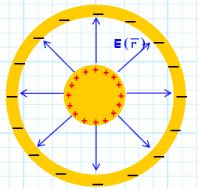We should note several things about these solutions: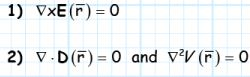3) Dand Eare normal to the surface of the conductor (i.e., their tangential components are equal to zero).

4)  The electric field is precisely the same as that given by eq. 4.31 in section 4-5!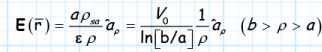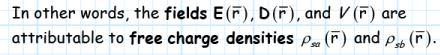Offer running on EduRev: Apply code STAYHOME200 to get INR 200 off on our premium plan EduRev Infinity!

159 docs

,

,

,

,

,

,

,

,

,

,

,

,

,

,

,

,

,

,

,

,

,

,

,

,

,

,

,

;